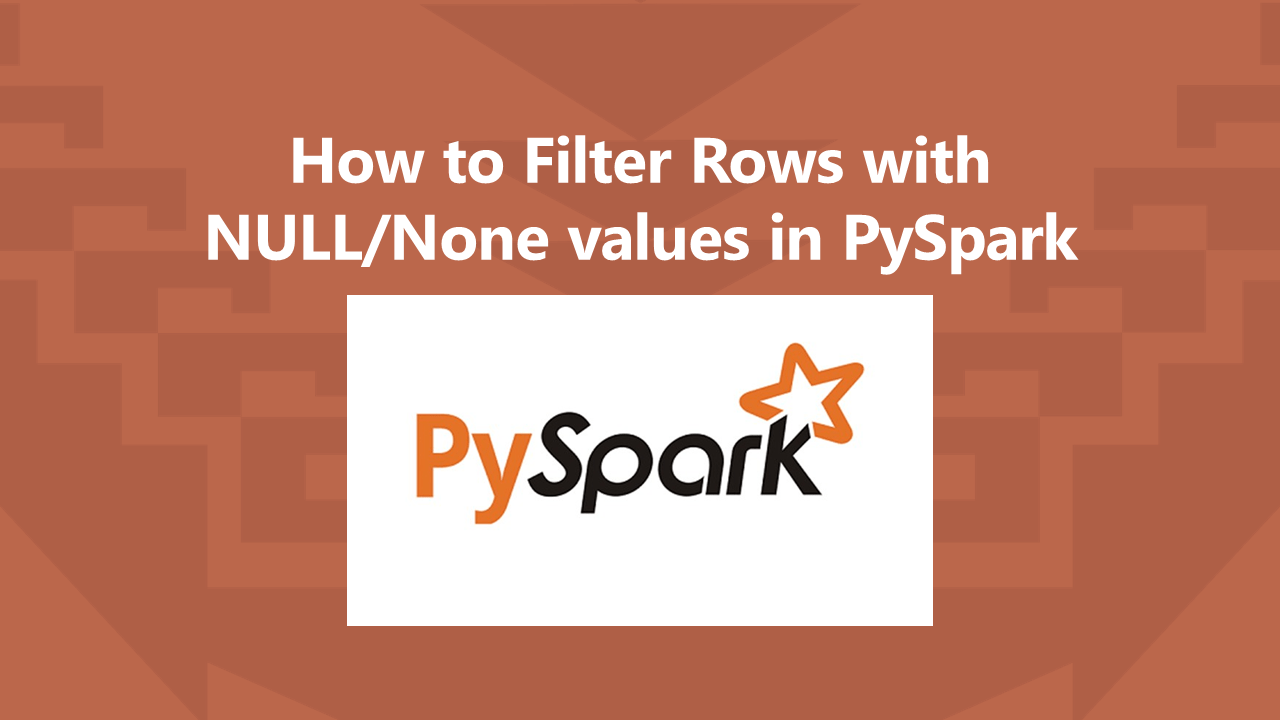Search

# PySpark How to Filter Rows with NULL ValuesWhile working on PySpark SQL DataFrame we often need to filter rows with NULL/None values on columns, you can do this by checking `IS NULL` or `IS NOT NULL` conditions.

In many cases NULL on columns needs to handles before you performing any operations on columns as operations on NULL values results in unexpected values.

Note: PySpark doesn’t support `column === null`, when used it returns an error.

We need to graciously handle null values as the first step before processing. Also, While writing DataFrame to the files, it’s a good practice to store files without NULL values either by dropping Rows with NULL values on DataFrame or By Replacing NULL values with empty string.

Before we start, Let’s create a DataFrame with rows containing NULL values.

``````
from pyspark.sql import SparkSession
spark = SparkSession.builder
.master("local")
.appName("SparkByExamples.com")
.getOrCreate()

data = [
("James",None,"M"),
("Anna","NY","F"),
("Julia",None,None)
]

columns = ["name","state","gender"]
df = spark.createDataFrame(data,columns)
df.show()
``````

This yields the below output. As you see I have columns `state` and `gender` with NULL values.

``````
+-----+-----+------+
| name|state|gender|
+-----+-----+------+
|James| null|     M|
| Anna|   NY|     F|
|Julia| null|  null|
+-----+-----+------+
``````

Now, let’s see how to filter rows with null values on DataFrame.

## Filter Rows with NULL Values in DataFrame

In PySpark, using `filter()` or `where()` functions of DataFrame we can filter rows with NULL values by checking `IS NULL` or `isNULL`.

``````
df.filter("state is NULL").show()
df.filter(df.state.isNull()).show()
df.filter(col("state").isNull()).show()
``````

These removes all rows with null values on `state` column and returns the new DataFrame. All above examples returns the same output.

``````
+-----+-----+------+
|name |state|gender|
+-----+-----+------+
|James|null |M     |
|Julia|null |null  |
+-----+-----+------+
``````

## Filter Rows with NULL on Multiple Columns

Let’s see how to filter rows with NULL values on multiple columns in DataFrame. In order to do so you can use either AND or && operators.

``````
df.filter("state IS NULL AND gender IS NULL").show()
df.filter(df.state.isNull() & df.gender.isNull()).show()
``````

Yields below output.

``````
+-----+-----+------+
|name |state|gender|
+-----+-----+------+
|Julia|null |null  |
+-----+-----+------+
``````

## Filter Rows with IS NOT NULL or isNotNull

`IS NOT NULL` or `isNotNull` is used to filter rows that are NOT NULL in PySpark DataFrame columns.

``````
from pyspark.sql.functions import col
df.filter("state IS NOT NULL").show()
df.filter("NOT state IS NULL").show()
df.filter(df.state.isNotNull()).show()
df.filter(col("state").isNotNull()).show()
``````

Yields below output.

``````
+----+-----+------+
|name|state|gender|
+----+-----+------+
|Anna|NY   |F     |
+----+-----+------+
``````

Alternatively, you can also write the same using `df.na.drop()`

``````
df.na.drop(subset=["state"]).show()
``````

## PySpark SQL Filter Rows with NULL Values

If you are familiar with PySpark SQL, you can check IS NULL and IS NOT NULL to filter the rows from DataFrame.

``````
df.createOrReplaceTempView("DATA")
spark.sql("SELECT * FROM DATA where STATE IS NULL").show()
spark.sql("SELECT * FROM DATA where STATE IS NULL AND GENDER IS NULL").show()
spark.sql("SELECT * FROM DATA where STATE IS NOT NULL").show()
``````

## Complete Example

Below is a complete Scala example of how to filter rows with null values on selected columns.

``````
from pyspark.sql import SparkSession
from pyspark.sql.functions import col
spark: SparkSession = SparkSession.builder
.master("local")
.appName("SparkByExamples.com")
.getOrCreate()

data = [
("James",None,"M"),
("Anna","NY","F"),
("Julia",None,None)
]

columns = ["name","state","gender"]
df =spark.createDataFrame(data,columns)

df.printSchema()
df.show()

df.filter("state is NULL").show()
df.filter(df.state.isNull()).show()
df.filter(col("state").isNull()).show()

df.filter("state IS NULL AND gender IS NULL").show()
df.filter(df.state.isNull() & df.gender.isNull()).show()

df.filter("state is not NULL").show()
df.filter("NOT state is NULL").show()
df.filter(df.state.isNotNull()).show()
df.filter(col("state").isNotNull()).show()
df.na.drop(subset=["state"]).show()

df.createOrReplaceTempView("DATA")
spark.sql("SELECT * FROM DATA where STATE IS NULL").show()
spark.sql("SELECT * FROM DATA where STATE IS NULL AND GENDER IS NULL").show()
spark.sql("SELECT * FROM DATA where STATE IS NOT NULL").show()
``````

## Conclusion

In this PySpark article, you have learned how to filter rows with NULL values from DataFrame/Dataset using IS NULL/isNull and IS NOT NULL/isNotNull. These come in handy when you need to clean up the DataFrame rows before processing.

Thanks for reading. If you recognize my effort or like articles here please do comment or provide any suggestions for improvements in the comments sections!

Happy Learning !!

#### Barbara Cartland

You should write because you love the shape of Flow and sentences and the creation of different words on a page.

####Subscribe